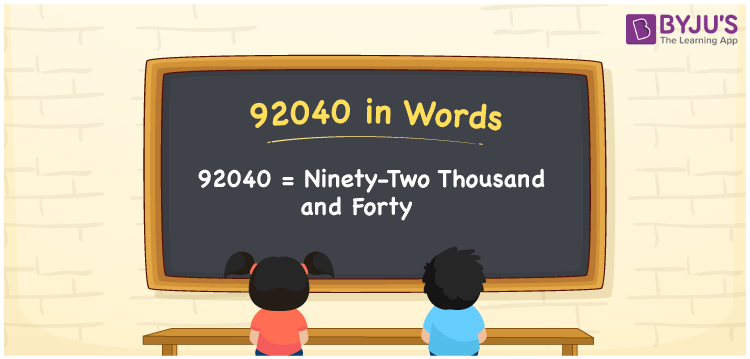# 92040 in Words

Ninety-two thousand and forty is the number name of 92040. For example, if you saved Rs. 92040 in 6 months, it can be written as “I saved Rs. Ninety-two thousand and forty”. Usually, the conversion from numbers to words is performed using the place value system. Now, let us discuss the procedure of writing the number 92040 in words in this article.

 92040 in Words: Ninety-two Thousand and Forty. Ninety-two Thousand and Forty in Numerical Form: 92040.

## 92040 in English Words## How to Write 92040 in Words?

The place values of 92040 are provided in the below table.

 Ten-thousands Thousands Hundreds Tens Ones 9 2 0 4 0

The expanded form of 92040 is as follows:

= 9 × Ten thousand + 2 × Thousand + 0 × Hundred + 4 × Ten + 0 × One

= 9 × 10000 + 2 × 1000 + 0 × 100 + 4 × 10 + 0 × 1

= 90000 + 2000 + 40

= 92040

= Ninety-two thousand and forty

Hence, 92040 in words is ninety-two thousand and forty.

92040 in words – Ninety-two thousand and forty

Is 92040 an odd number? – No

Is 92040 an even number? – Yes

Is 92040 a perfect square number? – No

Is 92040 a perfect cube number? – No

Is 92040 a prime number? – No

Is 92040 a composite number? – Yes

## Frequently Asked Questions on 92040 in Words

### Write 92040 in words.

92040 in words is ninety-two thousand and forty.

### Simplify 92000 + 40, and express it in words.

Simplifying 92000 + 40, we get 92040. Hence, 92040 in words is ninety-two thousand and forty.

### Is 92040 a composite number?

Yes, 92040 is a composite number.